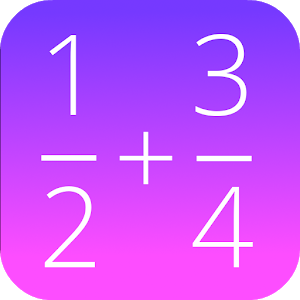# Fractions Math ProRating: 4.4/5

This calculator is the pro version of ‘Fractions Math’, and completely without advertisement.

This math app is a fractions calculator with various functions:

– add, subtract, multiply and divide fractions.
– convert fractions in decimals,
– simplify fractions
– compare fractions
– find the lowest common denominator
– fraction trainer, to learn fraction calculations